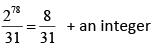Courses

# Test: Binomial Theorem - 1

## 20 Questions MCQ Test Topic-wise Tests & Solved Examples for IIT JAM Mathematics | Test: Binomial Theorem - 1

Description
This mock test of Test: Binomial Theorem - 1 for Mathematics helps you for every Mathematics entrance exam. This contains 20 Multiple Choice Questions for Mathematics Test: Binomial Theorem - 1 (mcq) to study with solutions a complete question bank. The solved questions answers in this Test: Binomial Theorem - 1 quiz give you a good mix of easy questions and tough questions. Mathematics students definitely take this Test: Binomial Theorem - 1 exercise for a better result in the exam. You can find other Test: Binomial Theorem - 1 extra questions, long questions & short questions for Mathematics on EduRev as well by searching above.
QUESTION: 1

### The sum of coefficients of (1 + x - 3x2)2134 is

Solution:

For the sum of coefficient, put x = 1, to obtain the sum is (1 + 1 - 3)2134 = 1

QUESTION: 2

### The sum rCr + r+1Cr + r+2Cr + .....+ nCr (n > r) equals

Solution:

C(n, r)  + c(n -1, r)  +  C(n - 2, r) +  ...  + C(r, r)
= r+1Cr+1 + r+1Cr + r+2Cr + .... +  n-1Cr + nCr
= n+1Cr+1   (applying same rule again and again )        (∴ nCr + nCr-1 = n+1Cr)

QUESTION: 3

### The expansion [x2 + (x6 - 1)1/2]5 + [x2 -(x6 - 1)1/2]5 is a polynomial of degree

Solution: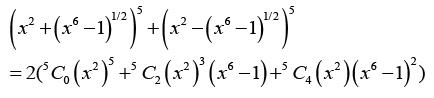Here last term   is of 14 degree.

QUESTION: 4

The term independent of x in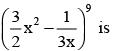Solution:

The general term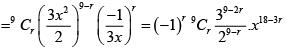The term  independent  of x, (or  the constant term) corresponds to x18-3r being  x0 or 18 - 3r = 0 ⇒ r = 6 .

QUESTION: 5

The value of the greatest term in the expansion of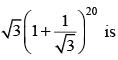Solution: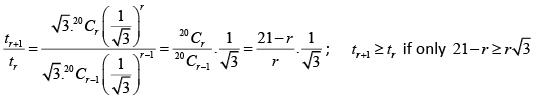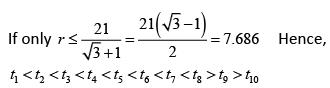Hence, t8 is the greatest term and its   value is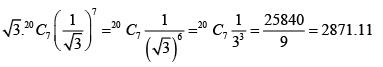QUESTION: 6

9n+1 - 8n- 9 is divisible by

Solution: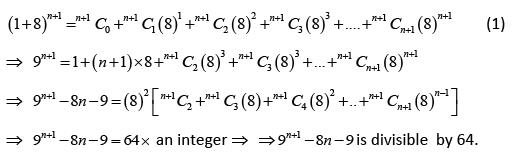QUESTION: 7

The first integral term in the expansion of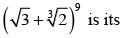Solution: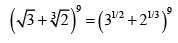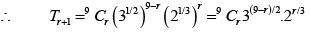For first integral term for r = 3;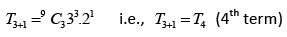QUESTION: 8

The number of irrational terms in the expansion of  (21/5 +31/10)55 is

Solution:

(21/531/10)55
Total terms = 55 + 1 = 56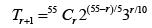Here r = 0, 10, 20, 30, 40, 50
Number of rational terms = 6;
Number of irrational terms = 56 - 6 = 50

QUESTION: 9

The number of terms in the expansion of (2x + 3y- 4z)n is

Solution:

We have, (2x + 3y - 4z)n = {2 + (3 - 4)}n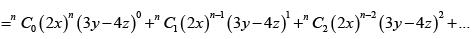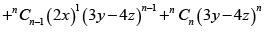Clearly, the first term in the above   expansion gives one term, second term gives two terms, third term gives three terms and so on.
So, Total  number of term = 1 +2+3+...+n+(n+1) =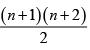QUESTION: 10

In the expansion of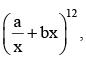the coefficient of x-10 will be

Solution:

Given expansion is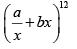∴  General term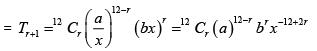Since, we have to find coefficient of x-10 ∴  -12 + 2r = -10  ⇒ r = 1
Now, then coefficient of x-10 is 12C1(a)11(b)1 = 12a11b

QUESTION: 11

If (1 + ax)n = 1 + 8x + 24x2 + ….., then the values of a and n are equal to

Solution: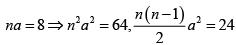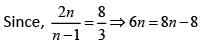∴ n = 4,a = 2

QUESTION: 12

The product of middle terms in the expansion of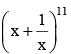is equal to

Solution: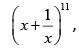it has 12 terms  in it’s  expansion , so there are two  middle  terms (6th and 7th);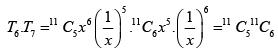QUESTION: 13

The middle term in the expansion of (1 – 2x + x2)n is

Solution: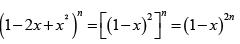Here 2n is even integer, therefore,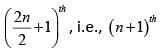term will be  the middle term.
Now, (n + 1)th term in (1 - x)2n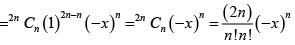QUESTION: 14

The sum of the  binomial coefficients in the expansion of (x-3/4 + ax5/4)n lies between 200 and 400 and the term independent of x equals 448. The value of a is

Solution: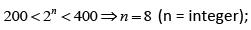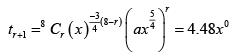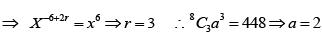QUESTION: 15

23C0 + 23C2 + 23C4 + ... 23C22 equals

Solution:

Given sum = sum of odd terms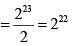QUESTION: 16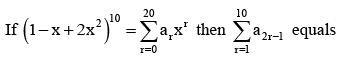Solution: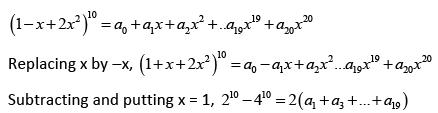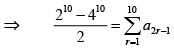QUESTION: 17

The greatest coefficient in the expansion of (1 + x)2n + 2 is

Solution:

Here  2n + 2 is even
Greatest  coefficient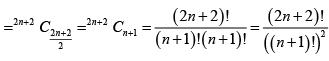QUESTION: 18

(nC0)2 + (nC1)2 + (nC2)2 + .....+ (nCn)2 equals

Solution: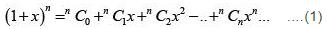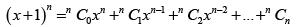Multiply (i) and (ii) and consider the coefficient x n of both sides, we have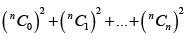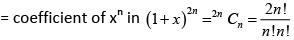QUESTION: 19

The value of C1 + 3C3 + 5C5 + 7C7 + ...., where C0, C3, C5, C7,..... are binomial coefficients is

Solution: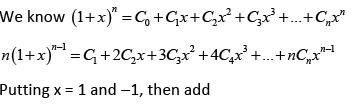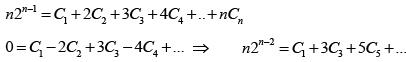QUESTION: 20

Fractional part of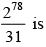Solution: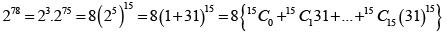278 = 8 + an integer multiple of 31;